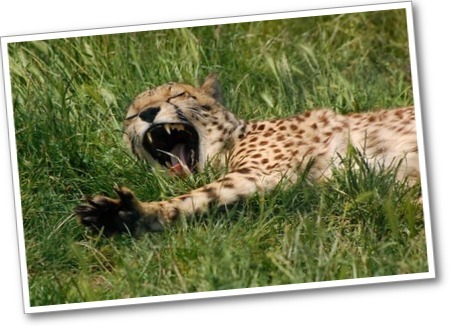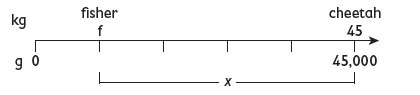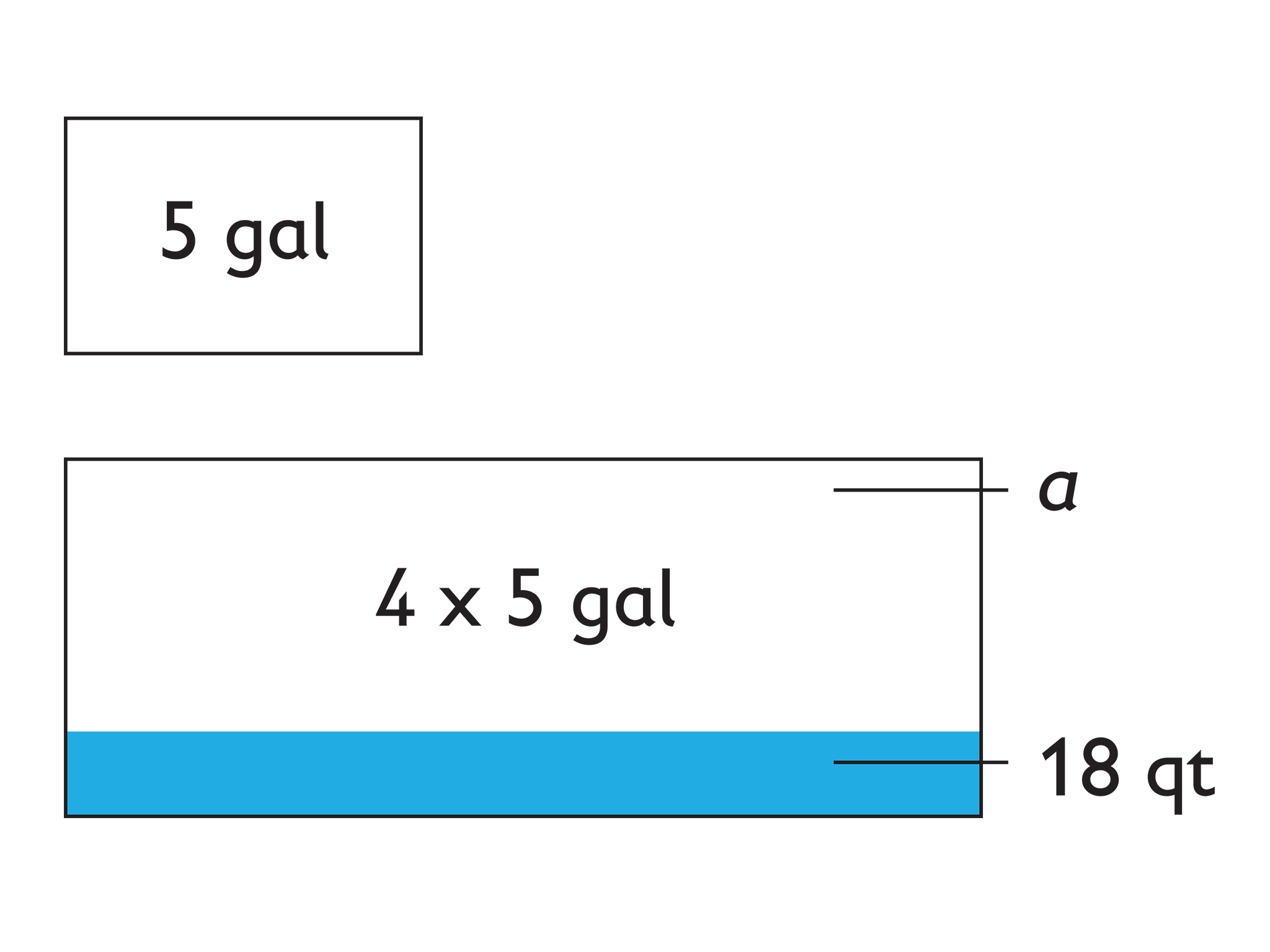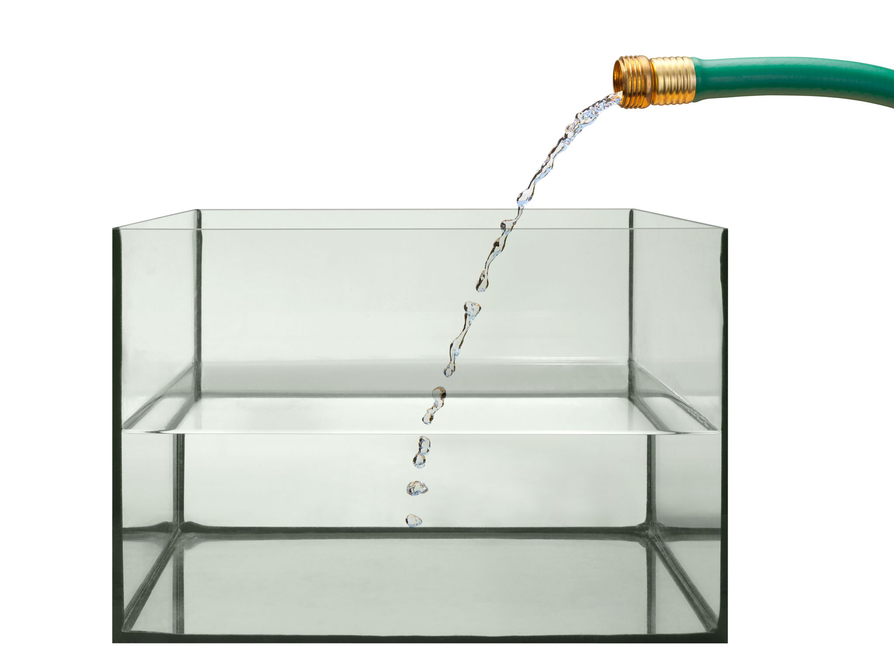Subject:
Numbers and Operations
Material Type:
Lesson Plan
Level:
Middle School
6
Provider:
Pearson
Tags:
Number Line, Problem Solving
Language:
English
Media Formats:
Text/HTML

# Gallery Problems# Gallery Overview

Allow students who have a clear understanding of the content thus far in the unit to work on Gallery problems of their choosing. You can then use this time to provide additional help to students who need review of the unit's concepts or to assist students who may have fallen behind on work.

# Gallery Description

Represent a Math Problem
Students explore the number line tool and the double number line tool. They use the number line tool to solve a problem about the weights of a cheetah and a fisher cat.

Research Expressions
Students learn the difference between numerical expressions and variable expressions. They watch video tutorials, review worked examples, use the Glossary, and explore other resources.

Fish Tank
Students create diagrams and use text and images as they solve a problem about the size of a fish tank.

# Represent a Math Problem

1. Students should explore number lines and double number lines.

• Students will select a representation to present to the class. See the work below for an example presentation.
2. Students will solve the problem and determine the difference between the mass of the cheetah and the fisher cat. See the work below for the solution.

3. No, Mia's answer does not make sense because the difference in the masses is a lot more than 36 g. 36 g is like 36 paper clips. The mass of the cheetah is 36,000 g more, not 36 g more. Here is how I checked Mia's answer. I used a number line:c = 45 kg = 5 × f

So, f = 45 kg ÷ 5 = 9 kg

x = c − f

So, x = 45 kg − 9 kg = 36 kg

36 kg = 36 × 1,000 g = 36,000 g

# Represent a Math Problem

1. Explore each of these math representations: a number line and a double number line.

• Select one representation to describe to the class.
2. Solve this problem. Use a number line or a double number line to help you solve it.

The cheetah at the zoo has a mass of 45 kg. This is 5 times the mass of the fisher cat.

• How many more grams is the mass of the cheetah than of the fisher cat?
3. The cheetah at the zoo has a mass of 45 kg. This is 5 times the mass of the fisher cat. How many more grams is the mass of the cheetah than of the fisher cat?

Mia says:

“The mass of the cheetah is 36 g more than the mass of the fisher cat.”

• Is Mia’s answer reasonable? Explain.

# Research Expressions

• Students should watch some of the tutorial videos found on the internet.
• Students should view some of the example problems.
• Students should look in the dictionary for a definition of expressions.
• Answers will vary. Sample answer: A numeric expression has numbers in it. A variable expression can contain numbers, but also has letters that represent unknown numbers.
• Answers will vary. Students should list five things they think they might learn about.
• Answers will vary. Sample answer: It is useful to research a concept when the concept is new to you and you don't know a lot about it.

# Research Expressions

Research to learn about the difference between a numerical expression (such as 2 + 3) and a variable expression (such as x + 2).

• Search using a few different keywords to find resources. Follow any links that look useful.
• Watch video tutorials about expressions.
• Review worked examples of expression problems.
• Look up expression in the dictionary.

• How is a numeric expression different from a variable expression?
• What are five things you think you will learn about expressions this year?
• When is it useful to research a concept?

# Fish Tankn = number of gallons of water the new tank holds

q = number of quarts of water the new tank holds

w = number of quarts of water in the tank

a = number of quarts more of water needed to completely fill the tank

n = 4 × 5 gal = 20 gal

q = 20 × 4 qt = 80 qt

a = qw

a = 80 qt − 18 qt = 62 qt

# Fish Tank

Anna has a 5 gal fish tank. She buys a new fish tank. It holds 4 times as much water as her old fish tank. Anna adds 18 qt of water to the new fish tank.

• Create a diagram to represent the situation.
• If Anna wants the new tank to be completely filled, how many more quarts of water does she need to add?